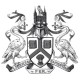# Mathematics Challenge

For a chance to win the first prize (£100 Amazon voucher), or one of our prizes for runners-up, submit your typed or neatly written solutions of the following problems to maths@lincoln.ac.uk or by post to Mathematics Challenge, School of Mathematics and Physics, University of Lincoln, Lincoln, LN6 7TS. Please include your full name, postal address and email, as well as the name and address of your school. The closing date is 5 January, 2017. The competition is open to all young pre-university people in UK aged 16–18 years. It is not open to current university students. See full Terms and Conditions. The problems can also be downloaded from here.

1. Suppose that alloy A contains 40% gold by weight, and alloy B contains 25% gold. How much of each of these alloys should be taken to be melted together so as to produce 600 grams of alloy containing 30% gold?

2.In a rectangle$ABCD$, the perpendicular$BE$ dropped from the vertex$B$ onto the diagonal$AC$ divides$AC$ in the ratio$1:3=AE:EC$. Find the length of$BC$ given the length$AB=1\,{\rm cm}$.

3. Solve the inequality$\displaystyle \frac{1}{3^x+1} \ + \ \frac{1}{3^x-3} \ \leq \ 0$.

4. Given an arbitrary convex quadrangle$ABCD$, each side of it is divided by two points in the ratio$1:\sqrt{2}:1$. Then a new quadrangle$PQRS$ is formed by constructing straight lines through pairs of these points as shown in the picture. Prove that the areas of the quadrangles$ABCD$ and$PQRS$ are equal.5. Prove that, given any 11 integers, one can choose some of them and put signs$+$ or$-$ between these chosen integers in such a way that the resulting sum is divisible by 2016.

(Recall that an integer$m$ is divisible by an integer$n$ if there is an integer$k$ such that$m=kn$; in particular,$0$ is also divisible by$n$.)

6. Given$2016$ non-zero real numbers, one can perform the following operation: replace any two of these numbers$a$ and$b$ by the numbers$(a-b)/2$ and$(a+b)/2$. Prove that one cannot return to the initial set of numbers after several such steps.

Notes: Full solutions are required – not just answers – with complete proofs of any assertions you may make. A winning submission may not necessarily be based on all six problems – so you are encouraged to submit solutions even if you do only some of the problems.

1.Evgeny Khukhro says:

Reblogged this on Algebra in Lincoln.

Like

1.Winners of the Mathematics Challenge 2016-17 – Maths & Physics News says: